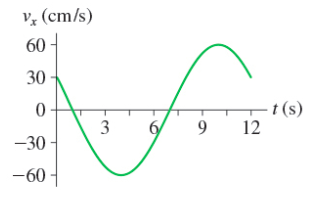# Problem: Part A. What is the amplitude of the oscillation? Express your answer to three significant figures and include the appropriate units.Part B. What is the phase constant? Express your answer in radians as positive number. Express your answer to three significant figures. Part C. What is the position at t = 0 s? Express your answer to three significant figures and include the appropriate units.

###### FREE Expert Solution

Velocity:

$\overline{){\mathbf{v}}{\mathbf{\left(}}{\mathbf{t}}{\mathbf{\right)}}{\mathbf{=}}{\mathbf{-}}{\mathbf{\omega }}{\mathbf{As}}{\mathbf{i}}{\mathbf{n}}{\mathbf{\left(}}{\mathbf{\omega }}{\mathbf{t}}{\mathbf{+}}{\mathbf{\varphi }}{\mathbf{\right)}}}$

Part A

Amplitude is the maximum displacement from the mean position.

In this case, the maximum velocity is 60 m/s

A = vmax/ω

91% (66 ratings)###### Problem DetailsPart A. What is the amplitude of the oscillation? Express your answer to three significant figures and include the appropriate units.